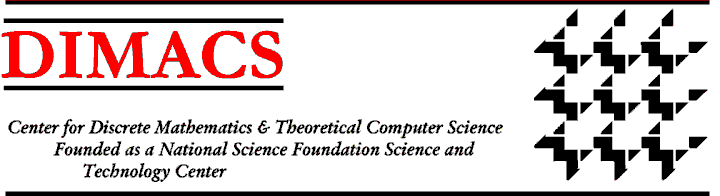### DIMACS Workshop on Feasible Arithmetics and Length of Proofs

#### April 21 - 23, 1996 Rutgers University, DIMACS Center, Piscataway, NJ

Organizers:
Paul Beame, University of Washington, beame@cs.washington.edu
Sam Buss, University of California - San Diego, sbuss@cs.ucsd.edu
Presented under the auspices of the DIMACS Special Year on Logic and Algorithms

This workshop will cover two complementary approaches to the complexity of proving a mathematical fact, with emphasis on their connections to circuit complexity and computational complexity. Feasible theories of arithmetic are weak first- or second-order systems whose theorems correspond to problems in computational classes such as the levels of the polynomial time hierarchy or the NC hierarchy. The ``length of proofs'' approach, on the other hand, studies the growth rate (in number of symbols, number of lines, logical depth, etc.) of proofs of instances of some theorem within a fixed, quantifier-free proof system.

Proof complexity and feasible arithmetics are closely related to each other and to fundamental problems from circuit complexity and computational complexity, such as the NP versus coNP problem. Over the last decade there has been significant progress in the study of these areas and many deep connections between them have been discovered. One example is the application of methods from circuit complexity lower bound proofs to obtain new lower bounds on proof complexity. Another example is the recent work on natural proofs, which has shown that if certain theories of bounded arithmetic can prove lower bounds in complexity theory, then certain levels of cryptographic security cannot be achieved.

The workshop will emphasize the interconnections among proof complexity, arithmetic complexity and computational complexity. This encompasses a broad range of topics of current interest, including Frege systems, bounded depth proof systems, cutting plane and Nullstellensatz proof systems, switching lemmas, recursion theoretic characterizations of complexity classes, bounded arithmetic and other feasible formal systems, cryptographic conjectures, interpolation theorems, independence results, interpretability and conservativity.Next: Call for ParticipationIndexDIMACS Homepage
Contacting the Center